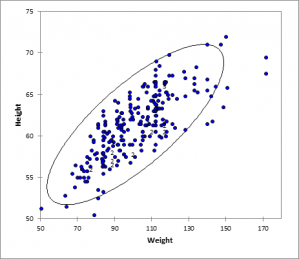# Scatter plots

Scatter plots are a simple way to visualize the association between two quantitative variables. Do it in Excel using the XLSTAT statistical software.## What is a scatter plot

A scatter plot or scattergraph is a type of diagram using Cartesian coordinates to display values for two or three variables for a set of data. The data is displayed as a collection of points, each having:

1. The value of one variable determining the position on the horizontal axis,
2. The value of the other variable determining the position on the vertical axis,
3. The value of the third determining the size of the point.
4. In addition a forth information can be added by using a qualitative variable to give the color to the points.

## Use of scatter plots

A scatter plot can suggest various kinds of correlations between variables.

The Scatter Plots tool allows you to save a lot of time when avoiding manipulating the Excel graphics to reach a satisfactory result. It can take into consideration:

• the possible belonging of an observation to a group,
• the possible superimposition of some points on the graphic,
• the possible presence of observation labels,
• the need to cross several X variables (abscissa) and Y variables Y (ordinates).### analyze your data with xlstat

14-day free trial